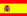You are here : > Home> Utilities > Financial calculus > Effective Rate of Discount>

### Effective Rate of Discount

To calculate the effective rate (compound) of a discount, enter data: Face value or future value (FV), Present discounted value (PV) and Term in days.
To separate decimal place, use the decimal point. E.g. 25,152.47 enter 25152.47; The result is showed, automatically, by clicking on "Calculate". For the calculation is assumed commercial year.

 Effective Rate of Discount Face value: Redemption value: Term in days: Monthly effective rate: Quarterly effective rate: Semiannual effective rate: Annual effective rate: